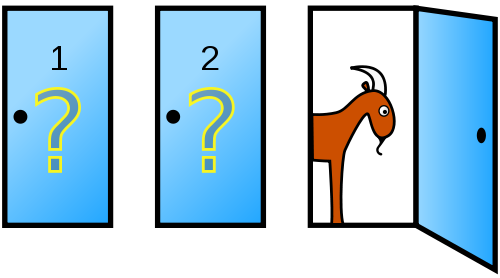I want to try to explain a really cool puzzle I found, which has caused confusion for decades.

The Monty Hall Problem is a famous probability puzzle which originated from a television show. In this show, a player is given the choice between three doors.

Behind one of these is a prize, a nice car, and the other doors contain goats. Upon picking a door, Monty Hall will reveal to you another door, which will always contain a goat.

He then asks you if you want to switch to the other, still unrevealed door. The question now is: does switching increase your chances of winning or not?Many say the odds remain of switching or not the same, but in reality it doesn’t. Chances of winning are effectively higher when switching doors.

Here is an example. Suppose you pick door number one. Out of the three doors the chance of any being the correct one is 1/3.

Let’s say Monty reveals to you door number three, which turns out to be a goat, rendering the chances of door three to be zero. Now, you might assume that the odds for door one and door two are 1/2. In reality, the probability of door two effectively shifts to door number two, which means that if you switch the chance is higher, 2/3, double of door one, speaking statistically.

If my explanation is not clear enough you should look at the table below and figure out yourself.

<th width="20%">
Door 2
</th>

<th width="20%">
Door 3
</th>

<th width="20%">
Result if not switching
</th>

<th width="20%">
Result if switching
</th>

<td width="20%">
<p align="left">
Goat
</p>
</td>

<td width="20%">
<p align="left">
Goat
</p>
</td>

<td width="20%">
<p align="left">
<b>Win</b>
</p>
</td>

<td width="20%">
<p align="left">
Lose
</p>
</td>

<td width="20%">
<p align="left">
<b>Car</b>
</p>
</td>

<td width="20%">
<p align="left">
Goat
</p>
</td>

<td width="20%">
<p align="left">
Lose
</p>
</td>

<td width="20%">
<p align="left">
<b>Win</b>
</p>
</td>

<td width="20%">
<p align="left">
Goat
</p>
</td>

<td width="20%">
<p align="left">
<b>Car</b>
</p>
</td>

<td width="20%">
<p align="left">
Lose
</p>
</td>

<td width="20%">
<p align="left">
<b>Win</b>
</p>
</td>

Door 1

Car

Goat

Goat

### Explanation with Mathematics

Let’s get some math involved here to clarify the solution.

We know that the probability for any door to be the correct pick is always constant, it is assumed the player picks door number one.The probability for the host to open door three is decided by the position of the car. The three cases are defined as following.

The first case, meaning that the player’s initial choice was correct. In this case the host could open door 2 or door 3.If door 2 is correct, meaning that the only door the host can open is the third one.If door 3 is correct, the host can’t reveal door 3.Following the event that the player chooses door one and the host opens door three, how big are the chances that door two is the correct one? We can use Bayes’ theorem to solve this problem.

It gives you the conditional probability for an event A giving that B is true.Now, in our case, we can calculate, meaning the conditional probability for door two to be the correct one (and so switching to be the correct choice) giving that we picked door one and the host opened door 3.is formed by adding all probabilities of the host opening door 3 in event that we pick door one, I described these earlier.Sincewe can simplify the equation toSo that’s the math explanation. If it’s not clear enough try to draw a tree diagram.